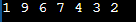# Inserting in a Circular Linked List in C

## Inserting a Node in a Circular Linked List in C Language with Examples:

In this article, I am going to discuss Inserting a Node in a Circular Linked List in C Language with Examples. Please read our previous article, where we discussed How to Create and Display Circular Linked List with Examples. At the end of this article, you will understand how to insert a node in a Circular Linked List with algorithm and examples using C Language.

##### Inserting a Node in a Circular Linked List:

In the linear linked list, we have learned the insertion process. So let us recall a few things from there. Here we have a linked list,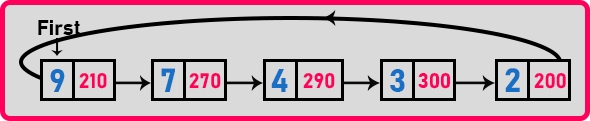This circular linked list contains 5 elements. Now let us label these nodes.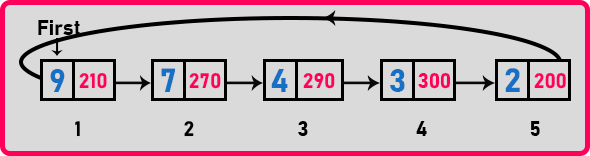We have given the indices to the nodes. Now, what are the positions that we have for inserting a new node in the above LinkedList? Let us mark those locations.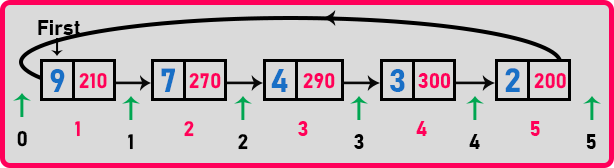Here pink number represents the node number and the black number represents the positions where we can insert a new node. So, we can insert before the first node which is the 0th position, we can insert between the nodes that are in the 1st, 2nd, 3rd, and 4th position and we can insert after the last node which is the 5th position. So, we have a total of 6 positions for insertion. We can insert a new node anywhere out of these 6 problems.

In the linear linked list, we had two cases. The first case is to insert before the first node and the second case is to insert at any other position in the linked list. Let us look at the second case first which is inserting at any other position.

##### Insert a Node at any other Position in a Linked List:

Suppose we want to insert a new node at the 4th position in the above LinkedList. First of all, we have to create a new node that is ‘t’ with some data,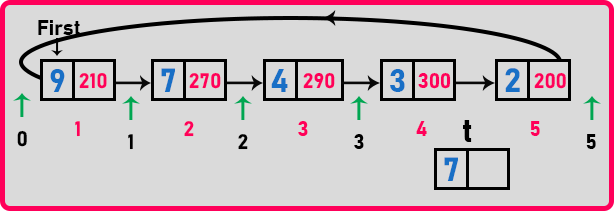For inserting the node ‘t’ at the 4th position, we should point the 4th node on ‘t’ and link the ‘t’ node to the 5th node. So, we have to change the 4th node also. For that, we need a pointer at the 4th node. We can bring a pointer from 1st node to 4th node as we have done in our previous articles.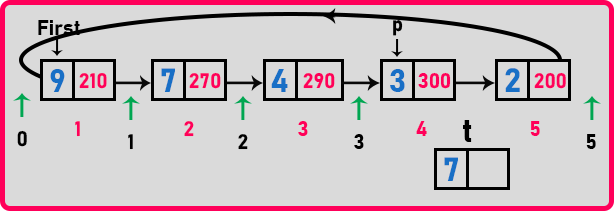Here ‘p’ pointer is pointing to the 4th node. Now we have to make t->next = p->next. So that ‘t’ will point to the 5th node.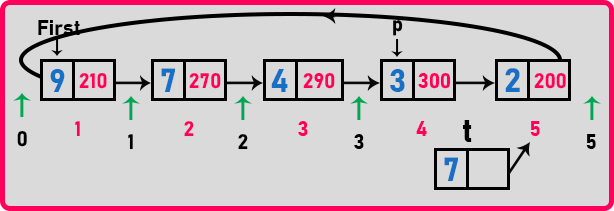Here ‘t’ is pointing to the 5th node. Now, instead of pointing to the 5th node, we have to point the ‘p’ node to the ‘t’ node.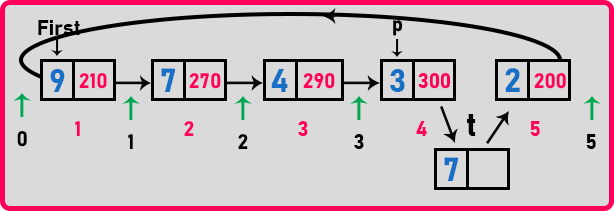Here ‘p’ node is pointing to the ‘t’ node and the ‘t’ node is pointing to the 5th node. This is the method of insertion for any other position in the linked list. This procedure is the same as what we have seen in inserting in the linear linked list.

##### Pseudo code for insertion at any position in a Linked List:

The pseudo-code for the insertion at any position is,

Node *t;

for(i = 0; i < pos-1; i++)
p = p->next;

t = new Node;
t->data = x;
t->next = p->next;
p->next = t;

We have already explained this code in our previous articles. If you don’t know what is happening, you can read the previous articles. This procedure takes minimum time when you want to insert just after the first node i.e. at 1st position in the linked list. It will take maximum time when you insert a new node after the last node.

So minimum time is constant time and the maximum time is of the order of n i.e. O (n). In the circular linked list, here is one position where we can insert in constant time that is after the first node. Now let us see the procedure of how to insert a new node before the head node.

##### Insertion a Node Before the Head Node in a Linked List:

Here also, we will create a new node that is ‘t’ and insert some data into it i.e. 7.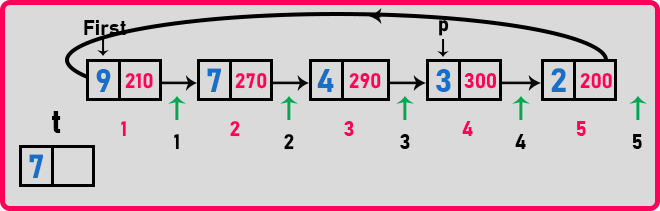Now t->next should point to the head or the first node. So,Here t->next is pointing to the first node. Now one more thing we have to do is that the last nodes next should point to the ‘t’ node.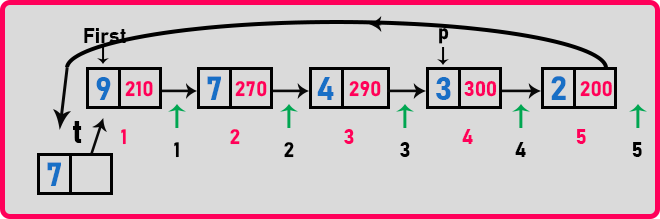Here we have changed or modified the last node to point to the t node. You can modify the last node by taking a pointer on the last node and changing it next to the ‘t’ node. As it is a circular linked list so on what basis we should stop at the last node? Because the last node is pointing at the head. So, we have to stop at that node whose next is pointing to the head or first node.

Now one important thing, should we move the head to node ‘t’? No. There is no need to shift the head pointer to the new node that is inserted before the head. So logically you don’t have to move the head pointer. You can move the head but it is not preferred. So, here, inserting before the head or inserting after the last node is the same. Below is the pseudo-code for the above procedure.

Node *t = new Node;
t->data = x;

p = p->next;

p->next = t;

We can call it inserting at the index 0th position. So, this is the procedure for inserting before the head node in the circular linked list. Now let us combine these two cases and create a single insert function for the circular linked list.

##### Complete Insert Function for Circular Linked list using C Language:
```void Insert (int pos, int x){
Node *t, *p;
int i;

if(pos == 0){
t = new Node;
t->data = x;
}
else{
p = p->next;
p->next = t;
}
}
else{
for(i = 0; i < pos - 1; i++)
p = p->next;
t = new Node;
t->data = x;
t->next = p->next;
p->next = t;
}
}
```

The insert function is taking two parameters which are position and the element that we want to insert. Then the first condition is that if the position is equal to 0 or before the head then the above-illustrated code will be executed. Already we have seen all the stuff. Then inside the else condition, insertion of the element with all the other indexes except 0 will take place. Now let us see the complete program.

##### Example to understand insertion in Circular Linked list using C Language:
```#include <stdio.h>
#include <stdlib.h>

struct Node {
int data;
struct Node * next;

void create(int A[], int n) {
int i;
struct Node * t, * last;
Head = (struct Node * ) malloc(sizeof(struct Node));

for (i = 1; i < n; i++) {
t = (struct Node * ) malloc(sizeof(struct Node));
t -> data = A[i];
t -> next = last -> next;
last -> next = t;
last = t;
}
}

void Display(struct Node * h) {
do {
printf("%d ", h -> data);
h = h -> next;

printf("\n");
}

void RDisplay(struct Node * h) {
static int flag = 0;

if (h != Head || flag == 0) {
flag = 1;
printf("%d ", h -> data);
RDisplay(h -> next);
}
flag = 0;
}
int Length(struct Node * p) {
int len = 0;
do {
len++;
p = p -> next;

return len;
}

void Insert(struct Node * p, int index, int x) {
struct Node * t;
int i;

if (index < 0 || index > Length(p))
return;

if (index == 0) {
t = (struct Node * ) malloc(sizeof(struct Node));
t -> data = x;
} else {
while (p -> next != Head) p = p -> next;
p -> next = t;
}

} else {
for (i = 0; i < index - 1; i++) p = p -> next;
t = (struct Node * ) malloc(sizeof(struct Node));
t -> data = x;
t -> next = p -> next;
p -> next = t;
}
}

int main() {
int A[] = {9, 7, 4, 3, 2};
create(A, 5);